# GeoPandas - spatial overlays, topology

## Geopandas 3¶¶In :
import geopandas as gpd
import matplotlib.pyplot as plt
%matplotlib inline


### Spatial overlays¶¶

Spatial overlays is the process of overlaying two or more layers on top of each other and performing operations based on how they overlay

#### Point in polygon problem¶¶

Geopandas is capable of higher level spatial overlay operations, but we can use shapely to perform low level geometry predicate operations as below:

In :
from shapely.geometry import Point, Polygon

p1 = Point(24.952242, 60.1696017)
p2 = Point(24.976567, 60.1612500)

# Create a Polygon
coords = [(24.950899, 60.169158), (24.953492, 60.169158), (24.953510, 60.170104), (24.950958, 60.169990)]
poly1 = Polygon(coords)

In :
gdf_points = gpd.GeoDataFrame(geometry=[p1,p2])
gdf_poly = gpd.GeoDataFrame(geometry=[poly1])

In :
fig, ax1 = plt.subplots()
gdf_poly.plot(ax=ax1)
gdf_points.plot(ax = ax1)

Out:
<matplotlib.axes._subplots.AxesSubplot at 0x11032d898>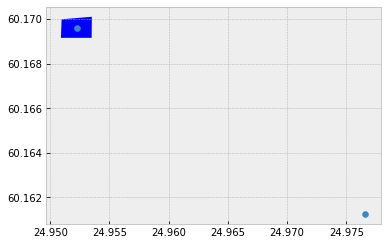Use the within() and contains() spatial topology operators

In :
p1.within(poly1)

Out:
True
In :
p2.within(poly1)

Out:
False
In :
poly1.contains(p1)

Out:
True

#### Topology inspections¶¶

In :
from shapely.geometry import LineString, MultiLineString

line_a = LineString([(0, 0), (1, 1)])
line_b = LineString([(1, 1), (0, 2)])

gdf_lines = gpd.GeoDataFrame(geometry=[line_a, line_b])
gdf_lines.plot(cmap='viridis')

Out:
<matplotlib.axes._subplots.AxesSubplot at 0x11041aac8>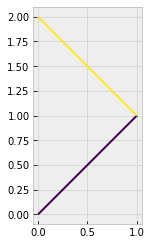In :
line_a.touches(line_b)

Out:
True
In :
line_a.intersects(line_b)

Out:
True

#### Spatial joins¶¶

the sjoin() is a powerful method. Internally it does the overlay operations and performs a spatial join.

In :
geocoded_address = gpd.read_file('./data/addresses_geocoded_3879.shp')

In :
fig3, ax3 = plt.subplots(1)
pop_poly.plot(ax=ax3)

Out:
<matplotlib.axes._subplots.AxesSubplot at 0x1a1bd5cf98>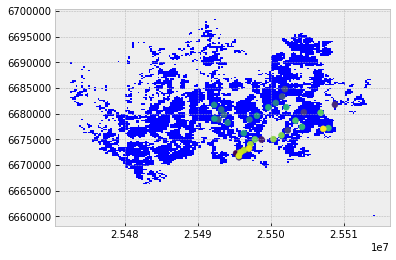Above you can see the polygons and the geocoded address points. Lets plot their dataframes to see the columns

In :
geocoded_address.head()

Out:
0 Itämerenkatu 14, 00180, Helsinki POINT (25495255.82235641 6672275.260963614)
1 Kampinkuja 1, 00100, Helsinki POINT (25496127.70873243 6672843.287593854)
2 Kaivokatu 8, 00100, Helsinki POINT (25496750.13674767 6673047.518228112)
3 Hermanstads strandväg 1, 00580, Helsingfors POINT (25498747.0563857 6674958.07898162)
4 Itäväylä 900, 00890, Helsinki POINT (25508815.55214499 6681781.436864837)
In :
pop_poly.head()

Out:
INDEX ASUKKAITA ASVALJYYS IKA0_9 IKA10_19 IKA20_29 IKA30_39 IKA40_49 IKA50_59 IKA60_69 IKA70_79 IKA_YLI80 geometry
0 688 8 31.0 99 99 99 99 99 99 99 99 99 POLYGON ((25472499.99532626 6689749.005069185,…
1 703 6 42.0 99 99 99 99 99 99 99 99 99 POLYGON ((25472499.99532626 6685998.998064222,…
2 710 8 44.0 99 99 99 99 99 99 99 99 99 POLYGON ((25472499.99532626 6684249.004130407,…
3 711 7 64.0 99 99 99 99 99 99 99 99 99 POLYGON ((25472499.99532626 6683999.004997005,…
4 715 19 23.0 99 99 99 99 99 99 99 99 99 POLYGON ((25472499.99532626 6682998.998461431,…

Rename a column

In :
pop_poly.rename(columns={'ASUKKAITA':'pop15'}, inplace=True)
pop_poly.columns

Out:
Index(['INDEX', 'pop15', 'ASVALJYYS', 'IKA0_9', 'IKA10_19', 'IKA20_29',
'IKA30_39', 'IKA40_49', 'IKA50_59', 'IKA60_69', 'IKA70_79', 'IKA_YLI80',
'geometry'],
dtype='object')

Filter and retain just the columns that are necessary. Then assert the CRS is same before doing spatial join

In :
pop_poly_filtered = pop_poly[['pop15', 'geometry']]

Out:
True

You specify the spatial operation when doing a join. Here the topology looks for within.

In :
geocoded_address_joined = gpd.sjoin(left_df=geocoded_address,
right_df=pop_poly_filtered,
how="inner", op='within')

Out:
0 Itämerenkatu 14, 00180, Helsinki POINT (25495255.82235641 6672275.260963614) 3214 521
1 Kampinkuja 1, 00100, Helsinki POINT (25496127.70873243 6672843.287593854) 3326 173
2 Kaivokatu 8, 00100, Helsinki POINT (25496750.13674767 6673047.518228112) 3449 31
11 Rautatientori 1, 00100, Helsinki POINT (25496939.39933316 6673189.887604699) 3449 31
4 Itäväylä 900, 00890, Helsinki POINT (25508815.55214499 6681781.436864837) 5680 28
In :
geocoded_address_joined.to_file('./data/address_geocoded_joined.shp')


Plot the data using classed colored renderer, based on the population column

In :
geocoded_address_joined.plot(column='pop15', cmap='Reds',
scheme='fisher_jenks', legend=True)
plt.title("Number of people living in the address' district")

Out:
Text(0.5,1,"Number of people living in the address' district")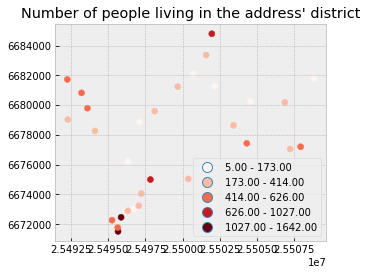### Spatial intersections¶¶

So far we saw how to join features based on their location, below we see how to intersect two layers and create new geometries.

Spatial joins preserve geometry and only get attributes. However, intersections and unions will create new (often smaller) geometries that include the characteristics of both the layers.

In :
borders_gdf = gpd.read_file('./data/helsinki_borders/Helsinki_borders.shp')


Out:
GML_ID NAMEFIN NAMESWE NATCODE geometry
0 27517366 Helsinki Helsingfors 091 POLYGON ((399936.363 6684600.244, 399937.63 66…
In :
travel_grid_gdf.head(3)

Out:
car_m_d car_m_t car_r_d car_r_t from_id pt_m_d pt_m_t pt_m_tt pt_r_d pt_r_t pt_r_tt to_id walk_d walk_t geometry
0 32297 43 32260 48 5785640 32616 116 147 32616 108 139 5975375 32164 459 POLYGON ((382000.0001358641 6697750.000038058,…
1 32508 43 32471 49 5785641 32822 119 145 32822 111 133 5975375 29547 422 POLYGON ((382250.0001358146 6697750.000038053,…
2 30133 50 31872 56 5785642 32940 121 146 32940 113 133 5975375 29626 423 POLYGON ((382500.0001357661 6697750.000038046,…
In :
travel_grid_gdf.shape

Out:
(13231, 15)
In :
# plot the boundary as basemap layer
basemap_ax = borders_gdf.plot(color='white', edgecolor='blue', figsize=(8, 12))

# do a class colored renderer on travel time by car column
travel_grid_gdf.plot(ax = basemap_ax, column='car_r_t',
alpha=0.5, cmap='plasma')

plt.tight_layout()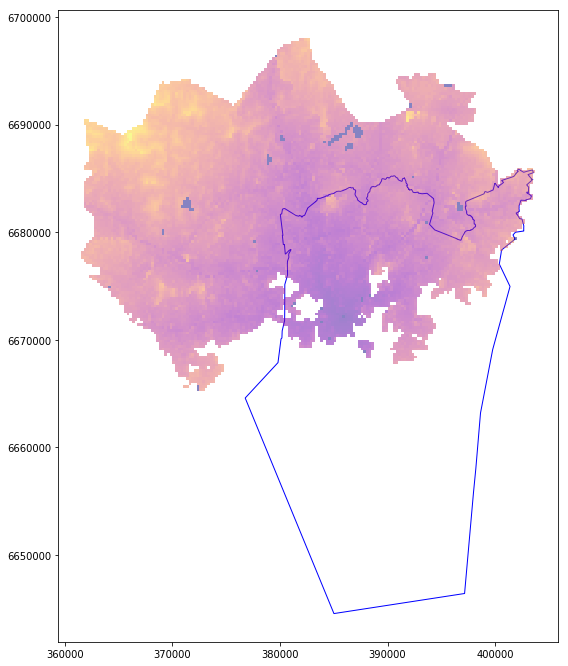The city of Helsinki is to the south. Thus we see the travel times increasing as we fan out. Now let us intersect the travel grid with basemap layer and select only the polygons that intersect

In :
%%time
intersect_result = gpd.overlay(travel_grid_gdf, borders_gdf, how='intersection')

CPU times: user 1min 18s, sys: 318 ms, total: 1min 18s
Wall time: 1min 19s


This is an expensive operations and is time consuming.

In :
intersect_result.head(4)

Out:
car_m_d car_m_t car_r_d car_r_t from_id pt_m_d pt_m_t pt_m_tt pt_r_d pt_r_t pt_r_tt to_id walk_d walk_t GML_ID NAMEFIN NAMESWE NATCODE geometry
0 15981 36 15988 41 6002702 14698 65 73 14698 61 72 5975375 14456 207 27517366 Helsinki Helsingfors 091 POLYGON ((391000.0001349226 6667750.00004299, …
1 16190 34 16197 39 6002701 14661 64 73 14661 60 72 5975375 14419 206 27517366 Helsinki Helsingfors 091 POLYGON ((390750.0001349644 6668000.000042951,…
2 15727 33 15733 37 6001132 14256 59 69 14256 55 62 5975375 14014 200 27517366 Helsinki Helsingfors 091 POLYGON ((391000.0001349143 6668000.000042943,…
3 15975 33 15982 37 6001131 14512 62 73 14512 58 70 5975375 14270 204 27517366 Helsinki Helsingfors 091 POLYGON ((390750.0001349644 6668000.000042951,…
In :
intersect_result.shape

Out:
(3836, 19)

Thus the number of records / polygons has reduced from around 13,000 to around 3800. Let us plot this to visualize the result

In :
intersect_result.plot()

Out:
<matplotlib.axes._subplots.AxesSubplot at 0x10dce8668>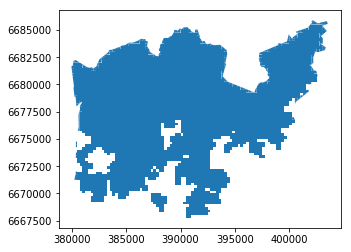#### Save as GeoJSON¶¶

The shape is similar to the shaded polygon in the previous plot. Let us save it to disk as a GeoJSON for future use.

In :
intersect_result.to_file('./data/travel_times/travel_times_overlay_helsinki.geojson',
driver='GeoJSON')


### Attribute aggregation¶¶

We can use regular pandas expressions to aggregate by attributes. For instance, we can aggregate all neighboring polygons grids that have the same travel time.

In :
intersect_result = gpd.read_file('./data/travel_times/travel_times_overlay_helsinki.geojson')

Out:
car_m_d car_m_t car_r_d car_r_t from_id pt_m_d pt_m_t pt_m_tt pt_r_d pt_r_t pt_r_tt to_id walk_d walk_t GML_ID NAMEFIN NAMESWE NATCODE geometry
0 15981 36 15988 41 6002702 14698 65 73 14698 61 72 5975375 14456 207 27517366 Helsinki Helsingfors 091 POLYGON ((391000.0001349226 6667750.00004299, …
1 16190 34 16197 39 6002701 14661 64 73 14661 60 72 5975375 14419 206 27517366 Helsinki Helsingfors 091 POLYGON ((390750.0001349644 6668000.000042951,…
2 15727 33 15733 37 6001132 14256 59 69 14256 55 62 5975375 14014 200 27517366 Helsinki Helsingfors 091 POLYGON ((391000.0001349143 6668000.000042943,…
In :
intersect_result['car_r_t'].value_counts()

Out:
 25    194
26    177
24    159
30    155
29    153
28    151
35    149
23    148
33    147
36    145
27    144
32    144
31    142
34    137
21    129
22    122
37    110
18    105
19    105
20    105
38     99
16     81
40     80
39     77
15     73
17     72
14     63
42     53
41     52
12     48
13     45
43     37
10     36
11     35
45     28
44     27
46     23
9      18
-1      12
47     12
8      10
48      7
51      6
7       5
50      5
49      5
56      2
53      1
52      1
54      1
0       1
Name: car_r_t, dtype: int64
In :
result_aggregated = intersect_result.dissolve(by='car_r_t')
result_aggregated.shape

Out:
(51, 18)
In :
result_aggregated.head(3)

Out:
geometry car_m_d car_m_t car_r_d from_id pt_m_d pt_m_t pt_m_tt pt_r_d pt_r_t pt_r_tt to_id walk_d walk_t GML_ID NAMEFIN NAMESWE NATCODE
car_r_t
-1 (POLYGON ((388000.0001354737 6669000.000042855… -1 -1 -1 5996387 -1 -1 -1 -1 -1 -1 -1 -1 -1 27517366 Helsinki Helsingfors 091
0 POLYGON ((386000.0001357812 6672000.000042388,… 0 0 0 5975375 0 0 0 0 0 0 5975375 0 0 27517366 Helsinki Helsingfors 091
7 POLYGON ((386250.0001357396 6671750.000042424,… 1059 7 1059 5977007 447 6 6 447 6 6 5975375 447 6 27517366 Helsinki Helsingfors 091
In :
result_aggregated.plot(cmap='plasma', figsize=(6,10), linewidth=0)

Out:
<matplotlib.axes._subplots.AxesSubplot at 0x10e37a6a0>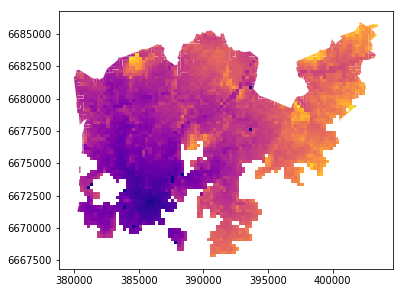Thus through aggregation, we have dissolved the number of features from 3800s to 51.

In :
result_aggregated.to_file('./data/travel_times/helsinki_aggregated.geojson',
driver='GeoJSON')